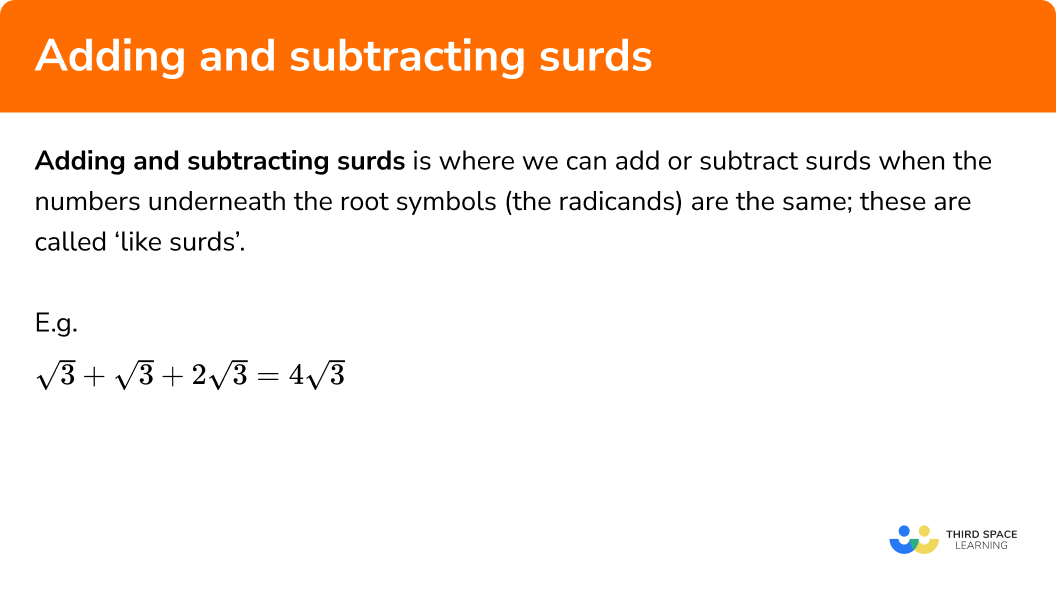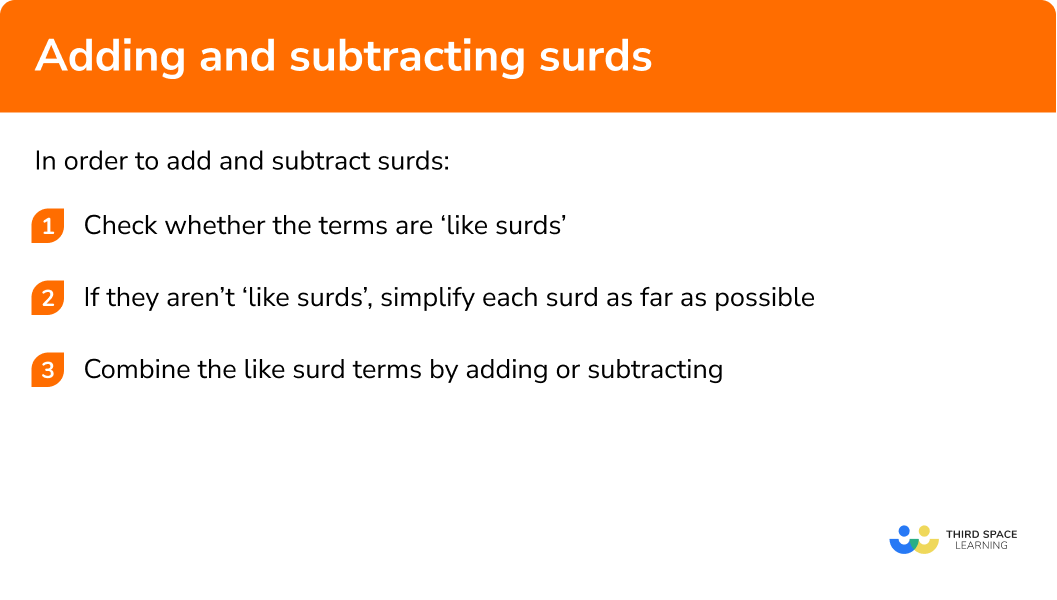GCSE Maths Number Surds

Here we will learn about adding and subtracting surds including when surd expressions can be added or subtracted, and how to carry out these calculations.

There are also adding and subtracting surds worksheets based on Edexcel, AQA and OCR exam questions, along with further guidance on where to go next if you’re still stuck.

## What is adding and subtracting surds?

Adding and subtracting surds is where we can add or subtract surds when the numbers underneath the root symbols (the radicands) are the same; these are called ‘like surds’.

This is similar to collecting like terms in algebra:

E.g.

a + a + 2a simplifies to 4a.

So when we do a similar thing with surds:

E.g.

$\sqrt{3}+\sqrt{3}+2\sqrt{3}=4\sqrt{3}$

And just as 2a+3b cannot be simplified, because a and b are not like terms:

2\sqrt{3}+3\sqrt{7} cannot be simplified because the numbers underneath the square root symbols (radicands) are different. \sqrt{3} and \sqrt{7} are not ‘like surds’.

If surds can be simplified so that they are ‘like surds’, then they can be added or subtracted.

You may be asked to apply these skills at GCSE maths to give answers to problems in geometry, such as Pythagoras or trigonometry, as exact values, rather than as decimals. Before calculators were invented, surds were the standard form for stating answers which were irrational numbers.

The formula for solving quadratics also uses a square root sign, so you may need to apply your knowledge of surds here as well.

### What is adding and subtracting surds?## How to add and subtract surds

In order to add and subtract surds:

1. Check whether the terms are ‘like surds’.
2. If they aren’t like surds, simplify each surd as far as possible.
3. Combine the like surd terms by adding or subtracting.

### How to add and subtract surds## Adding and subtracting surds examples

### Example 1: like surds, simple addition

Simplify

$2\sqrt{3}+5\sqrt{3}$

1. Check whether the terms are ‘like surds’.

The number under the root sign is already 3 in both terms, so they have a common radicand and are like surds.

2If they aren’t like surds, simplify each surd as far as possible.

We don’t need to change the surd terms in this question.

3Combine the like surd terms by adding or subtracting.

$2+5=7$

so,

$2\sqrt{3}+5\sqrt{3}=7\sqrt{3}$

### Example 2: like surds, simple subtractions

Simplify

$7\sqrt{5}-9\sqrt{5}+6\sqrt{5}$

Check whether the terms are ‘like surds’.

If they aren’t like surds, simplify each surd as far as possible.

Combine the like surd terms by adding or subtracting.

### Example 3: unlike surds

Simplify

$10 \sqrt{2}+4 \sqrt{11}$

Check whether the terms are ‘like surds’.

If they aren’t like surds, simplify each surd as far as possible.

Combine the like surd terms by adding or subtracting.

### Example 4: sums containing non-surds

Simplify

$4+6\sqrt{2}-3\sqrt{2}-\sqrt{25}$

Check whether the terms are ‘like surds’.

If they aren’t like surds, simplify each surd as far as possible.

Combine the like surd terms by adding or subtracting.

### Example 5: one surd needs to be simplified

Simplify

$\sqrt{7}+\sqrt{28}$

Check whether the terms are ‘like surds’.

If they aren’t like surds, simplify each surd as far as possible.

Combine the like surd terms by adding or subtracting.

### Example 6: both surds need to be simplified

Simplify

$\sqrt{8}+\sqrt{72}$

Check whether the terms are ‘like surds’.

If they aren’t like surds, simplify each surd as far as possible.

Combine the like surd terms by adding or subtracting.

### Example 7: two surds need to be simplified

Simplify

$\sqrt{75}+\sqrt{50}$

Check whether the terms are ‘like surds’.

If they aren’t like surds, simplify each surd as far as possible.

Combine the like surd terms by adding or subtracting.

### Example 8: a sum containing non-surds

Simplify

$\sqrt{90}+\sqrt{64}-\sqrt{40}$

Check whether the terms are ‘like surds’.

If they aren’t like surds, simplify each surd as far as possible.

Combine the like surd terms by adding or subtracting.

### Common misconceptions

• Remember that a root with no integer coefficient is ‘1 lot’ of that surd

As in algebra, we understand that a actually means 1a.

• Don’t mix up addition and multiplication laws

$\sqrt{3}+\sqrt{3}=2 \sqrt{3}$

$\sqrt{3} \times \sqrt{3}=\sqrt{9}=3$

Refer back to knowledge of algebra to help:

a + a = 2a and a × a = a2.

• Not simplifying each surd fully

As in example 6 above, if we don’t simplify each surd fully, it may look like the surds cannot be simplified to give ‘like surds’.

• Trying to combine unlike surds

It’s OK to leave an answer with more than one surd in it if it will not simplify further.

Adding and subtracting surds is part of our series of lessons to support revision on surds. You may find it helpful to start with the main surds lesson for a summary of what to expect, or use the step by step guides below for further detail on individual topics. Other lessons in this series include:

### Practice adding and subtracting surds questions

1. Simplify:

3 \sqrt{11}+2 \sqrt{11}-\sqrt{11}

6 \sqrt{11}4 \sqrt{11}7 \sqrt{11}5 \sqrt{11}Already in like surd form; 3+2-1=4 .

2. Simplify:

3\sqrt{5}-2\sqrt{3}+4\sqrt{3}

3 \sqrt{5}+2 \sqrt{3}5 \sqrt{15}-24 \sqrt{15}3 \sqrt{5}-2 \sqrt{3}We can only collect the ‘like’ root 3 s; 4-2=2 .

3. Simplify:

\sqrt{11}+\sqrt{44}

5\sqrt{11}\sqrt{55}3\sqrt{11}11 \sqrt{5}4 is a square factor of 44 , so use this to simplify root 44 . There’s then a like surd of root 11 .

4. Simplify:

\sqrt{54}-\sqrt{24}

\sqrt{30}6\sqrt{30}6\sqrt{6}\sqrt{6}9 is a square factor of 54 and 4 is a square factor of 24 . When you simplify both roots, there’s a like surd of root 6 .

5. Simplify:

3\sqrt{20}-\sqrt{50}

3\sqrt{1000}6-\sqrt{10} \sqrt{5}6\sqrt{5}-5\sqrt{2}6\sqrt{10} \sqrt{5}When both surds are fully simplified, they are not like surds. We just write the answer as the subtraction with simplified surds.

6. Simplify:

3 \sqrt{16}+\sqrt{50}-\sqrt{8}

12+5 \sqrt{10}-2 \sqrt{2}12+3 \sqrt{2}\sqrt{2}(3+3 \sqrt{8})12-3 \sqrt{2}Root 16 is not a surd, so we work out 3\times4=12 . Then, looking at the surds, when both are simplified, there is a like surd of root 2 . We combine these two surds.

### Adding and subtracting surds GCSE questions

1. Express \sqrt{6}+\sqrt{54} in the form a \sqrt{6} where a is an integer.

(2 marks)

\sqrt{54}=\sqrt{9} \times \sqrt{6}

(1)

\sqrt{6} + \sqrt{54} =4\sqrt{6} (a=4)

(1)

2. Simplify fully \sqrt{32}+\sqrt{2}

(2 marks)

\sqrt{32}=\sqrt{16} \times \sqrt{2}

(1)

5 \sqrt{2}

(1)

3. Write \sqrt{40}+\sqrt{160} in the form a \sqrt{10}

(3 marks)

\sqrt{40}=\sqrt{4} \times \sqrt{10}

(1)

\sqrt{160}=\sqrt{16} \times \sqrt{10}

(1)

\sqrt{40} + \sqrt{160} =6\sqrt{10} (a=6)

(1)

## Learning checklist

You have now learned how to: